Next: Round Robin Classification Up: Round Robin Classification Previous: Introduction   Contents

Class Binarization

Many machine learning algorithms are inherently designed for binary (two-class) decision problems. Prominent examples are perceptrons, support vector machines, the original ADABOOST algorithm, and separate-and-conquer rule learning. In addition, all regression algorithms can, in principle, be used for binary decision problems, but not for multi-class problems (unless, maybe, if the class values can be ordered). On the other hand, real-world problems often have multiple classes. Fortunately, there exist several simple techniques for turning multi-class problems into a set of binary problems. We will call such techniques class binarization techniques.

Definition 2.1 (class binarization, decoding, base learner)   A class binarization is a mapping of a multi-class learning problem to several two-class learning problems in a way that allows a sensible decoding of the prediction, i.e., it allows the derivation of a prediction for the multi-class problem from the predictions of the set of two-class classifiers. The learning algorithm used for solving the two-class problems is called the base learner.

The most popular class binarization technique is the unordered or one-against-all class binarization, where one takes each class in turn and learns binary concepts that discriminate this class from all other classes. It has been independently proposed for rule learning (Clark and Boswell, 1991), neural networks (Anand et al., 1995), and support vector machines (Cortes and Vapnik, 1995).

Definition 2.2 (unordered/one-against-all class binarization)   The unordered class binarization transforms a c-class problem into c two-class problems. These are constructed by using the examples of class i as the positive examples and the examples of classes j (j=1 ...c, j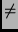i) as the negative examples.

The name ``unordered'' originates from Clark and Boswell (1991), who proposed this approach as an alternative to the decision-list learning approach that was originally used in CN2 (Rivest, 1987; Clark and Niblett, 1989). As our main concern is rule learning, we will primarily stick to the terminology used there, but will also occasionally refer to it as one-against-all, which seems to be predominant in other fields.

Definition 2.3 (ordered class binarization)   The ordered class binarization transforms a c-class problem into c-1 binary problems. These are constructed by using the examples of class i (i = 1 ...c-1) as the positive examples and the examples of classes j > i as the negative examples.

Figure: Unordered and round robin binarization for a 6-class problem.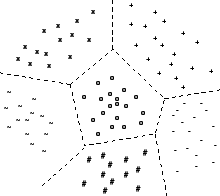(a) Multi-class learning one classifier that separates all classes.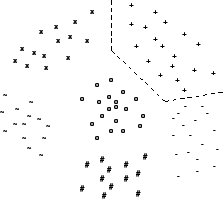(b) Unordered learning c classifiers, each separates one class from all other classes. Here: + against all other classes.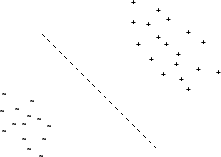(c) Round robin learning c(c-1)/2 classifiers, one for each pair of classes. Here: + against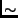.

Note that ordered class binarization imposes an order on the induced classifiers, which has to be adhered to at classification time: the classifier learned for discriminating class 1 from classes 2 ...c has to be called first. If this classifier classifies the example as belonging to class 1, no other classifier is called; if not, the example is passed on to the next classifier. Unordered class binarization, on the other hand, has to call each of its constituent binary classifiers and requires some external criterion for combining the individual predictions into a final prediction. Typical decoding rules vote the predictions of the individual classifiers, possibly by taking into account the confidences of the predictions (cf. Section 7).Next: Round Robin Classification Up: Round Robin Classification Previous: Introduction   Contents
Johannes Fürnkranz 2002-03-11